# Basic Techniques for Accurate Resistance Measurement

At CAS DataLoggers, calls are frequently received from people who work in resistance measurement applications, such as those who use string pots to measure movement, measuring thermistors/RTDs for temperature, measuring the resistance of test samples, as well as many other applications. Many of the callers are surprised to hear that there’s a range of different techniques that can be employed to realize more accurate measurements.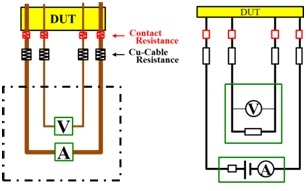CAS DataLoggers also converse with operators who report getting strange data points, such as: “With the logger I’m using, I’m seeing numbers that don’t make sense.” This is usually remedied by the realization that resistance measurements can be spread across many different ranges, which requires different measurement techniques to be used. In this white paper, we look at a few simple ways to lower the error and improve accuracy across the low, mid, and high resistance ranges.

## Use the Right Measurement Technique for Your Range

Resistance measurements are denoted by the ohm unit (Ω). 1 ohm is the resistance between two points of a conductor when a continuous potential difference of 1 volt is introduced to these points, and this produces a current of 1 ampere in the conductor, that is, if the conductor does not produce any of its own electromotive force.

Resistance is one of the few electronic values that varies over a huge range (over 12 orders of magnitude) across different applications, and many operators don’t consider this when collecting the data. For many applications, values below 100 ohms can be considered as the low resistance value, while between 100 ohms and 1,000,000 ohms (mega-ohm) is the intermediate range.

High resistance ranges start at the mega-ohm range and can go all the way up to the giga-ohm range (1 billion ohms). When callers ask, “I need to measure resistance—what logger do you recommend?” the Application Specialists will help them to narrow the choice down by asking, “What’s the resistance range you’re trying to measure? Milliohm, kilo-ohm?”

Before commencing with your application, it is important to realize that each of these ranges require different measurement techniques. In fact, there isn’t a single technique that can measure every resistance value, and it is very easy to obtain inaccurate results because of using the wrong technique for your application range.

For example, if you don’t match your technique with your range, your data may only be accurate to within 5% of the actual value. For some applications, this isn’t a major issue, but in other instances, your measurements will need to be significantly more accurate, such as when recording data at the milliohm level or when recording at giga-ohm levels– where the accuracy of the readings can significantly vary depending on the capacitance and insulation resistance of your cabling.

Out of the three, mid-range measurements are the simplest, whereas very low- and high-range measurements can bring about problems which are realized in the form of measurement errors and lower accuracies.

## Ohm’s Law

The fundamental principle of resistance measurement, Ohm’s Law, states that, the ratio of the potential difference (V) across the ends of a conductor of resistance (R) to the current (I), flowing in that conductor will be at a constant value when the temperature is also constant.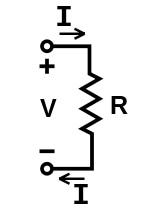For many applications you can use the basic Ohm’s Law formula of I = V/R, where I is the current through the conductor (amperes), V is the potential difference across the conductor (volts), and R is the resistance of the conductor (a constant with its unit in Ohms).

Ohm’s Law is simple to work with if you wish to find any of these values. For example, it is also correct that Resistance equals Voltage divided by Current (R=V/I), and that Voltage = Current multiplied by Resistance (V=I*R). Using this approach, you can deduce any missing variable if the other two values are known.

## Using Data Loggers for Resistance Measurement

As well as using multimeters, resistance can be determined using a correctly-configured universal input data logger. However, these dataloggers have a limited measurement range, so this must be considered. Universal analog inputs enable dataloggers to log voltage or current measurements, calculate the values of resistance and save the data.

In addition to the measurement instrument you choose to employ, there are subtleties about measuring resistance that you must understand to increase the accuracy of your measurements.

## Low-Resistance Measurement

Let’s first look at the cables that connect the device being measured to the instrument: If you’re measuring resistance close to the source, you will get different readings to those from a sensor located 200 ft away. For example, if we have a resistor which we wish to measure, the first step is to somehow connect it, so we connect a wire to a 1-ohm resistor.

However, the wire Is not an ideal conductor—it gives some resistance within itself, just like any piece of wire. Let’s say that this resistance is 1 ohm per 100 ft (a typical value for a 20-gauge wire), and we have 3 ft worth of cabling going to and from the device (6 ft. total), we would expect to obtain a resistance reading of 1 ohm, but our actual resistance value is 1.06 ohms.

When measuring smaller resistance values, a common method is to force a known current followed by measuring the voltage across the Device Under Test (DUT–see Figure 1 below). This is in adherence to Ohm’s Law since you’re backing out the resistance using the current and voltage. We are assuming that you have a precision current source (for example of 2 milliamps or 200 microamps), and a voltmeter with high accuracy.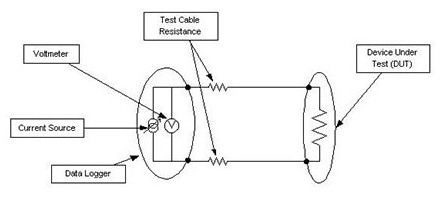Figure 1. Forcing a Known Current

You run two wires on both sides of the resistor, followed by forcing the current through both sets of leads. However, this will produce a measurement error because the voltage measured at the end of the leads will not be equal to the voltage across the resistor, because it also includes the voltage drop across the wires between the meter and DUT.

Therefore, in these cases you can minimize the error by performing a 4-wire measurement (see Figure 2 below) where one set of leads is used to carry the sourced current, and a second set of leads is only used to determine the voltage across the resistor.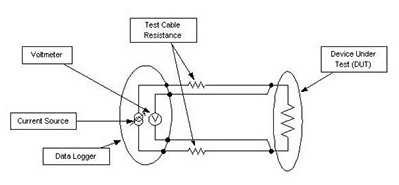Figure 2. Typical 4-Wire Measurement

This approach yields much more accurate results by navigating around the additional voltage drop induced by the current source through the leads. In this instance, it is assumed that the voltmeter draws a negligible current, and this is not usually a concern.

At CAS DataLoggers, the dataTaker and Grant Squirrel data loggers can perform 4-wire measurements for RTDs and other sensors. These loggers will force a current through a pair of wires followed by measuring the voltage from the other pair of wires. However, there is a limit to how large of a resistance can be recorded. For example, around the 10,000 Ohm range, the smallest amount of current that can be forced by the data logger is 200 μamps, and this results in a voltage of 2 volts.

At higher resistance values (for example, 1 million ohms) this current would produce a voltage of 200 volts– a value well beyond the logger’s capabilities. It’s not possible to use 4-wire measurement methods for measuring high values of resistance using these data logger types, as you require the source to either produce a very small current or measure a large voltage.

When measuring a low resistance, it is feasible to use an alternative approach to the 4-wire measurement by removing one of the conductors and undertaking a 3-wire measurement. In this approach, you measure two voltages: the voltage across the resistor and the voltage across the conductor carrying the test current.

After performing these measurements, you can back out the error as there is a measurable voltage drop in one of the leads. When the error has been deduced, just double it and subtract it from your measurements to obtain a more accurate reading. Most data loggers can perform this 3-wire measurement, including the dataTaker data loggers and the Grant Squirrel series mentioned above.

While using the 3-wire measurement saves you a piece of wire, it does assume that the voltage drop in the two leads is identical. Unfortunately, this is can often, but not always, be the case. If the voltage drop is not even, then you will be privy to errors when using this approach.

While many operators are often measuring in the 0 to 100,000 Ohm range, there are specialized sensors, such as conductivity or test samples, that fall within very high resistance ranges; so, it may be the case that you have to adopt a different technique at high resistances:

## High Resistance Measurement

For recording a high resistance, you can employ a reliable technique that is the inverse of the one detailed above for measuring low resistances. In this approach, a voltage is forced, and the current is measured so that the resistance be calculated (again in accordance with Ohm’s Law). There are a few ways that you can perform this method:

The first method needs a high-precision instrument which is designed to measure at very low currents. If your voltage source and current meter have a negligible resistance, you can force 5 volts through the meter connected to the DUT and record the current. For example, if the resistance is a million ohms (1 mega-ohm), the current would be relatively small at 5 μA.

A different method for measuring large resistances is to employ a voltage source in series, alongside a known test resistance that will stimulate the unknown resistance, followed by measuring the voltage across the test resistance (see Figure 3 below). With the value of the test voltage source already known, alongside the known resistance and the voltage across this resistance (including Ohm’s Law and bit of algebra), enables you to determine the unknown resistance: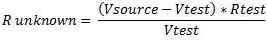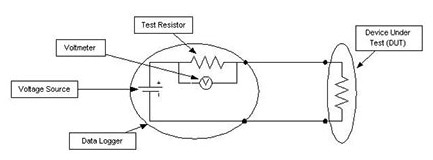Figure 3. Sourcing Voltage Through a Known Resistor

For this technique to be effective, the value of the test resistance needs to be within one or two orders of magnitude of the unknown resistance. Again, your voltmeter will need to have a good accuracy, otherwise an error will be present in your measurements. Additionally, the voltmeter used to measure Vtest should not load the circuit, i.e. its input impedance should be around 100 to 1,000 times greater than Rtest.

Another problem with performing high resistance measurements, is that, even when insulated cables are used, no insulation is 100% perfect—there is always some form of current leakage. For example, there could be 10 million ohms of resistance between the center of the wire and the surface it is placed on, so the current leakage could affect your measurement in an adverse manner.

To minimize this, you can employ a technique known as ‘guarding’, which uses a shielded cable alongside a separate voltage source which goes into the shields and around the cables. The separate voltage source is used to apply the same voltage to the shield.

In this approach, even though there’s a resistance between the central conductor and the shield, they are maintained at the same voltage. This means that there is no leakage of the current because a separate voltage source was used to guard it. This technique works well but needs a meter that has been designed to provide a guard voltage or a second voltage source.

When a high-range resistance is being measured, you will find that you can drastically reduce your measurement errors by employing one of the above methods.

## Intermediate Resistance Measurements

Within the intermediate range, the employed technique will ultimately depend on the measuring device being used—there’s no one method that can be relied on. Luckily, errors are less frequent, and measurements are more direct within measuring within the intermediate resistance range.

## Summary

At resistance values below 10,000 ohms, you can employ the techniques above for the low resistance ranges. When the values are at 100 ohms and lower, it is imperative to obtain accurate readings. Similarly, at values above 100,000 ohms, i.e. high resistance ranges, it is best to employ the force voltage technique as described above.

Basically, when obtaining resistance measurements, you will need to understand whether you’re in a high or low resistance range and apply the appropriate techniques– as this will help to minimize, or completely negate any large errors from occurring and give you much higher measurement accuracy.This information has been sourced, reviewed and adapted from materials provided by CAS DataLoggers.

## Citations

• APA

CAS DataLoggers. (2019, July 16). Basic Techniques for Accurate Resistance Measurement. AZoSensors. Retrieved on July 20, 2019 from https://www.azosensors.com/article.aspx?ArticleID=1318.

• MLA

CAS DataLoggers. "Basic Techniques for Accurate Resistance Measurement". AZoSensors. 20 July 2019. <https://www.azosensors.com/article.aspx?ArticleID=1318>.

• Chicago

CAS DataLoggers. "Basic Techniques for Accurate Resistance Measurement". AZoSensors. https://www.azosensors.com/article.aspx?ArticleID=1318. (accessed July 20, 2019).

• Harvard

CAS DataLoggers. 2019. Basic Techniques for Accurate Resistance Measurement. AZoSensors, viewed 20 July 2019, https://www.azosensors.com/article.aspx?ArticleID=1318.

## Tell Us What You Think